Next: Refraction
Up: EXPLANATION AND EXAMPLES
Previous: Mean Place to Apparent Place

## Apparent Place to Observed Place

The observed place of a source is its position as seen by a perfect theodolite at the location of the observer. Transformation of an apparent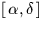to observed place involves the following effects:

•to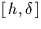.
• Diurnal aberration.
•to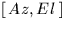.
• Refraction.
The transformation from apparentto apparentis made by allowing for Earth rotation through the sidereal time,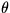: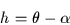For this equation to work,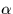must be the apparent right ascension for the time of observation, andmust be the local apparent sidereal time. The latter is obtained as follows:
1.
from civil time obtain the coordinated universal time, UTC (more later on this);
2.
add the UT1-UTC (typically a few tenths of a second) to give the UT;
3.
from the UT compute the Greenwich mean sidereal time (using sla_GMST);
4.
add the observer's (east) longitude, giving the local mean sidereal time;
5.
add the equation of the equinoxes (using sla_EQEQX).
The equation of the equinoxes (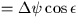plus small terms) is the effect of nutation on the sidereal time. Its value is typically a second or less. It is interesting to note that if the object of the exercise is to transform a mean place all the way into an observed place (very often the case), then the equation of the equinoxes and the longitude component of nutation can both be omitted, removing a great deal of computation. However, SLALIB follows the normal convention and works via the apparent place.

Note that for very precise work the observer's longitude should be corrected for polar motion. This can be done with sla_POLMO. The corrections are always less than about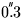, and are futile unless the position of the observer's telescope is known to better than a few metres.

Tables of observed and predicted UT1-UTC corrections and polar motion data are published every few weeks by the International Earth Rotation Service.

The transformation from apparentto topocentricconsists of allowing for diurnal aberration. This effect, maximum amplitude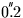, was described earlier. There is no specific SLALIB routine for computing the diurnal aberration, though the routines sla_AOP etc. include it, and the required velocity vector can be determined by calling sla_GEOC.

The next stage is the major coordinate rotation from local equatorial coordinatesinto horizon coordinates. The SLALIB routines sla_E2H etc. can be used for this. For high-precision applications the mean geodetic latitude should be corrected for polar motion.Next: Refraction
Up: EXPLANATION AND EXAMPLES
Previous: Mean Place to Apparent Place

SLALIB --- Positional Astronomy Library